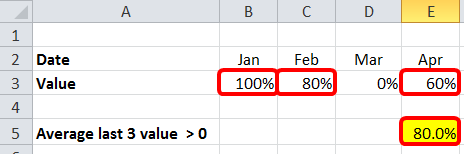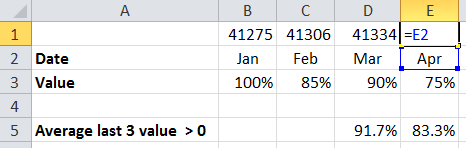# Formula Forensics No. 035 Average the last 3 values greater than 0

A couple of weeks ago Amanda asked a question at Chandoo.org

“I need to calculate a moving average of the last 3 months.

However, if one of the months contains 0%, I want it to ignore that and take the last month that didn’t have a zero.

For instance, my data is this: April = 100%, May=0%, June=97%,July=98%, August=0%.

My formula is only looking at June July and August, but since August has a 0 I would like it to look at May June and July.

And since May has a 0, I would like it to look at April, June and July.”

I offered a solution which is an array formula.

=AVERAGE(AVERAGEIFS(\$B\$3:F3,\$B\$2:F2,LARGE(IF(\$B\$3:F3>0,\$B\$2:F2),{1,2,3}))) Ctrl+Shift+Enter

Today I am going to try and explain what it is doing and how it works.

## The Problem

How do we write a formula to extract the last 3 non zero values?

What if there is more than 1 zero value?

What if the zero values are non-contiguous?

This can all be shown by:

Average of the last 3 records – No ZeroesAverage of the last 3 records – One Zero in Current MonthAverage of the last 3 records – One Zero  in a Previous MonthAverage of the last 3 records – Multiple Zeroes## A Solution

=AVERAGE(AVERAGEIFS(\$B\$3:F3,\$B\$2:F2,LARGE(IF(\$B\$3:F3>0,\$B\$2:F2),{1,2,3}))) Ctrl+Shift+Enter

Normally at Formula Forensics we start in the inside of a formula and work out, but today we are going to start at the outside and work our way in.

The solution: =AVERAGE(AVERAGEIFS(\$B\$3:F3,\$B\$2:F2,LARGE(IF(\$B\$3:F3>0,\$B\$2:F2),{1,2,3})))

This function is the Average() of an Averageifs() function.

What is going on here you ask ?

We know that the Average() function will average the constituent numbers. eg: =Average(6, 8, 10) = 8

But doesn’t Averageifs return a single number? The average of its components!

We’ll mostly, but not always.

Lets have a look:

If we remove the outside average and just look at the inner Averageifs() function

In the sample file, Cell F15 you will see:

=AVERAGEIFS(\$B\$3:F3,\$B\$2:F2,LARGE(IF(\$B\$3:F3>0,\$B\$2:F2),{1,2,3})) Press F2 then F9,

Excel evaluates this as:

={0.5,0.9,0.7}

So the Averageifs() function is returning 3 values being 0.5, 0.9 & 0.7

These are the last 3 values greater than 0 ranked from latest to earliest by date

Which is exactly what Amanda asked us to average

So we will need to look inside the Averageifs() function to see what is going on.

The Syntax for the Averageifs() function is:

AVERAGEIFS(average_range, criteria_range1, criteria1, [criteria_range2, criteria2], …)

in our example

Average_range : \$B\$3:F3 This is the Values we want to average from teh start of the data up to the current cell

Criteria_range1 : \$B\$2:F2 This is the Date Range from the start of teh data up to the current cell

Criteria1 : LARGE(IF(\$B\$3:F3>0,\$B\$2:F2),{1,2,3}) This is a function that will return the 3 largest values shown by the array {1,2,3}

So in in English, the Averageifs function is being asked to return a single value from the Value row where the Date row matches the largest, second largest and third largest criteria. In each case Averageifs will average the number but as it is a single number it returns the value by itself.

Lets now step into the Large() function and see what is going on.

LARGE(IF(\$B\$3:F3>0,\$B\$2:F2),{1,2,3})

The Large() function has the syntax =Large(Array, k)

In our example:

Array: IF(\$B\$3:F3>0,\$B\$2:F2)

k: {1,2,3} This is an array and hence asks for the Largest (1), Second largest (2) and Third Largest (3) values

So we are getting the 3 largest values from the formula: IF(\$B\$3:F3>0,\$B\$2:F2)

What is the IF(\$B\$3:F3>0,\$B\$2:F2) formula doing?

If you put the formula IF(\$B\$3:F3>0,\$B\$2:F2)

into a blank cell say F31 and press F9

Excel returns: {41275,41306,41334,41365,FALSE}

These are the date values of the date row up to the Column we are working in

You can see these in Row 1 of the sample fileYou will notice that there is a False value in the position of the Column which has the Value of 0%

This is derived by the If() Function.

The If() function is saying: IF(\$B\$3:F3>0,\$B\$2:F2)

If the Value Row >0 (\$B\$3:F3>0), return the Date Row (\$B\$2:F2) else return False

The False isn’t shown in the formula, it is returned by Default when a False argument doesn’t exist

The standard Syntax for If is =If(Criteria, Value when true, Value when false)

In our case we don’t have a Value when false component and so Excel simply places a false in as the answer.

Applying the formula using Ctrl+Shift+Enter forces Excel to Evaluate the formula as an Array Formula

What this means in practice is that the Formulas are evaluated 3 times as per the array {1,2,3} effectively extracting the last 3 values that match the last 3 dates where the Value is >0

## What if I want to Average a Different Number of Days?

You have two choices

### 1. Change the Array Manually

If say you want to average the previous 5 values

You can modify the array manually

=AVERAGE(AVERAGEIFS(\$B\$3:F3,\$B\$2:F2,LARGE(IF(\$B\$3:F3>0,\$B\$2:F2),{1,2,3,4,5}))) Ctrl+Shift+Enter

This is ok if it is not done regularly or is only slightly different to the existing array.

But if you want to setup the top twenty you need to type {1,2,3,4,5,6,7,8,9,10,11,12,13,14,15,16,17,18,19,20}

Which is quite clumsy!

Bad Hint: You can copy the array from above.

Or you can automate it using the technique below.

### 2. Insert a Formula to Setup the Array

You can add a small function that will automatically setup the array like:

=AVERAGE(AVERAGEIFS(\$B\$3:F3,\$B\$2:F2,LARGE(IF(\$B\$3:F3>0,\$B\$2:F2),ROW(OFFSET(\$A\$1,,,F39,1))))) Ctrl+Shift+Enter

This assume that cell F39 has a number which is the number of periods you want to average

You can read more about how the ROW(OFFSET(\$A\$1,,,F39,1)) part works in previous Formula Forensics posts

## Other Posts in this Series

The Formula Forensics Series contains a wealth of useful solutions and information.

## Two Challenges

### 1. Can you solve this another way

Just after I posted my solution, Chandoo posted an alternative solution which you can read at:

http://chandoo.org/wp/2009/04/28/calculate-moving-average/#comment-446559

Can you solve this problem another way ?

Let us know in the comments below:

If you have a clever formula and would like to become an author here at Chandoo.org please consider writing it up as I have done above. Alternatively you can send the formula to either Chandoo or Hui.Hello Awesome...

My name is Chandoo. Thanks for dropping by. My mission is to make you awesome in Excel & your work. I live in Wellington, New Zealand. When I am not F9ing my formulas, I cycle, cook or play lego with my kids. Know more about me.

Thank you and see you around.

### Related articles:

 Written by Hui... Tags: average(), averageifs, if(), large Home: Chandoo.org Main Page ? Doubt: Ask an Excel Question

### 17 Responses to “Formula Forensics No. 035 Average the last 3 values greater than 0”

1.Sajan says:

Hi Hui,
Nice write up!

Here is one more approach, as an array formula:
=AVERAGE(LOOKUP(LARGE(IF(Values,COLUMN(Values)),{1,2,3}), COLUMN(Values), Values))

Cheers,
Sajan.

2.Jason M says:

=SUM(G4:INDEX(rng,LARGE(IF(rng>0,COLUMN(rng)-MIN(COLUMN(rng))+1),3)))/3

Array-entered.

3.Jason M says:

Or take out the hard-coded G4 and go with:

=SUM(INDEX(rng,MAX(COLUMNS(rng))):INDEX(rng,LARGE(IF(rng>0,COLUMN(rng)-MIN(COLUMN(rng))+1),3)))/3

Array-entered.

4.Andrew says:

Here's one that lets you specify the number of values to average in a cell (AvgNum):

=SUMPRODUCT((COLUMN(Values)>=LARGE(COLUMN(Values)*(Values>0),AvgNum))*(Values))/AvgNum

•Andrew says:

Forgot to say that as this is SUMPRODUCT it doesn't need to be array entered.

Also rather rather than using the date values to discern the order it assumes oldest to most recent is left to right, if you wanted right to left then replace the >=LARGE with <=SMALL, and if the values were vertical then swap the COLUMN formulas for ROW.

I opted for this way as all you need are the values, they don't need to be linked to dates, and it doesn't need to be in a table or formatted any particular way.

•Randy says:

Thanks for the formula!

5.Narayana Murthy says:

This trick is really great. Actually i came to know about the array formula through this post. I have hooked to array formula for the past three days .. have read nearly all the posts on array formula on your site... 🙂

6.Micah Dail says:

=AVERAGEIFS(tbl[Value], tbl[Date],">"&LARGE(((tbl[Value]0)*tbl[Date]),4),tbl[Value],"0")

That's my solution. I took the liberty of adding the data to a table in order to standardize it. One can easily change the "4" in the formula to adjust the range of the moving average.

•Micah Dail says:

I just realized that I was missing the "" in the formula I typed in. Please see updated formula. As with others in this thread, this is array-entered. Also, to clarify what I posted, changing the "4" in the formula to a referenced cell will make short work of adjusting the moving average range.

`=AVERAGEIFS(tbl[Value], tbl[Date],">"&LARGE(((tbl[Value]0)*tbl[Date]),4),tbl[Value],"gt.lt 0")`
swap gt.lt for Not Equal codes

•Micah Dail says:

Well it seems that the comments do not accept a "less than greater than" [does not equal] notation. In the formula, just insert this before the "0" within the quotations.

Sorry about the spamming of the comment section; I just wanted to clarify my formula.

7.zurman says:

Array formula are truly handy

8.??? says:

9.??? says:

“=SUM((LARGE(IF(\$B\$3:D3,\$B\$2:D2),{1;2;3})=\$B\$2:D2)*\$B\$3:D3)/3?
ctrl+shift+enter

10.dannny says:

“=SUM((LARGE(IF(\$B\$3:D3,\$B\$2:D2),{1;2;3})=\$B\$2:D2)*\$B\$3:D3)/3
ctrl+shift+enter

11.Ankit says:

This is nice:)

12.Adam says:

I have a column that i need the last moving average of the last 21 rows that are not 0 averaged. But i also need it to get the average even if there is only 1 cell that isn't 0. So it would be

D3 - Average
D4:D87 data that gets updated daily.

I am not sure how to get this to work.

•Hui... says:

Try: =AVERAGE(OFFSET(INDIRECT("\$D"&MAX((\$D\$4:\$D\$87>0)*ROW(\$D\$4:\$D\$87))),,,-21,1)) Ctrl+Shift+Enter

 « PowerPivot – the ULTIMATE anti-bloat feature Chandoo.org produces it’s Second MVP. »

### Get FREE Excel & Power-BI Newsletter

One email per week with Excel and Power BI goodness. Join 100,000+ others and get it free.